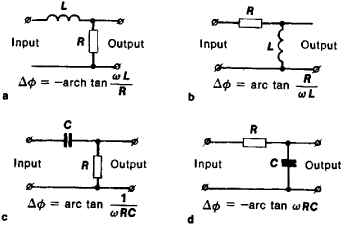# Phase-Shift Circuit

## phase-shift circuit

[′fāz ¦shift ‚sər·kət]
(electronics)
A network that provides a voltage component which is shifted in phase with respect to a reference voltage.

## Phase-Shift Circuit

an electric circuit in which the oscillation phases of the individual harmonic spectrum components of the propagated signal at the output of the circuit differ from the phases of the corresponding components at the input of the circuit. In phase-shift circuits with lumped parameters, the phase shift is due to the effects of the reactive components, such as capacitors and induction coils. In phase-shift circuits with distributed parameters, the phase shift is due to the finite time required for the propagation of the electric signal from the input to the output of the circuit.Figure 1. Elementary phase-shift circuits consisting of L networks: (L) induction coil, (R) resistor, (C) capacitor; (Δφ) phase shift, (ω) angular frequency

The simplest phase-shift circuits consist of four-terminal L networks containing either a reactive component and a resistor or two reactive components of different kinds (Figure 1). Such circuits are usually used to introduce a fixed phase shift into a transmission channel carrying signals at frequencies specified for mains used by industry or at radio frequencies (up to the meter wavelength range). Phase-shift circuits are of great practical importance in radio engineering. They are used to change the wave form of input signals (in differentiating and integrating devices), to compensate for phase distortion, and to produce phase modulation.

### REFERENCE

Zernov, N. V., and V. G. Karpov. Teoriia radiotekhnicheskikh tsepei, 2nd ed. [Leningrad] 1972.

K. K. TOVARA

Site: Follow: Share:
Open / Close